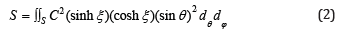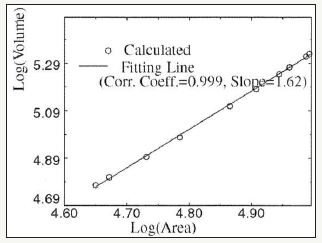• Submissions# Analysis of Feng, Sitek and Gullberg’s model for the Surface Area of the Left Ventricle

José Sérgio Domingues1*, Vale MP2 and Martinez3

1 Department of Mathematics, Federal Institute of Minas Gerais, Brazil

2 Cardiovascular Surgery, Nossa Senhora das Dores Hospital, Brazil

3 Department of Mechanical Engineering, Federal University of Minas Gerais, Brazil

*Corresponding author: José Sérgio Domingues, Department of Mathematics, Federal Institute of Minas Gerais (IFMG), Formiga, MG, Brazil

Submission: February 02, 2018;Published: August 31, 2018

DOI: 10.31031/OJCHD.2018.02.000541ISSN 2578-0204
Volume2 Issue4

#### Mini Review

In 2001, a mathematical model for determining the surface area of the left ventricle (LV) and another one for its volume (VLV) was presented . Both were based on the consideration that the geometry of a normal LV is similar to the one of a Truncated Prolate Spheroid (TPS) [2-6]. The ellipsoidal coordinates were considered, which are harder to obtain than linear coordinates, which are easily achievable with echocardiographic exams (Figure 1).

figure 1:Geometric representation of the LV with elliptical coordinates .The goal was to verify if there was a strong linear relationship between the VLV and the surface area of the LV, and in case this relationship was proven, the VLV could be estimated based on its surface area. From this geometric model, two equations were obtained: one for the VLV, and another one for its surface area (Equations 1 and 2).where J is the determinant of the Jacobian matrix of the variables x, y, and z. The obtained results show a near perfect linear correlation between log (Volume) and log (Area), which can be seen on Figure 2. However, only the data of 10 patients were used, and their features were not taken into account, such as gender, age, body surface, height, etc. This lack of description of the patients’ specific features precludes a more detailed analysis of this parameter.

figure 2:Correlation between VLV and the area of the LV .Besides, the VLV was calculated by considering the same model that was used on determining the surface area of the LV, that is, the volume of a TPS was calculated and, later, the surface area of the same TPS. That way, mathematically, we can already expect a perfect correlation, because the calculated parameters are closely related to the model that was used, making them dependent on each other.

This dependence between the equations for area and volume was reviewed for average data of the diastolic diameter of the LV on asymptomatic Brazilian population, on the work of [7,8]. However, in this project, he used Cartesian mathematical models, because they facilitated its practical application, since the variables that are needed for determining the VLV and its surface area (Equations 3 and 4) are linear.In these equations, a and c represent the smallest and biggest semi-axes of the truncated spheroid, and |𝜎| and 𝑥(𝑧) represent the truncating point, and the equation on the positive part of the truncated spheroid’s curve. By performing the simulations and determining the linear correlation between the VLV and the surface area of the LV, it is possible to notice, as in , the direct dependency between these two parameters, that is 𝑟=0.9999. This happens due to the fact that all the equations (Equations 1-4) are based on the same geometry for the LV (Figure 3).

figure 3:Correlation between VLV and the area of the LV for the Cartesian model.#### Conclusion

Therefore, it is unrealistic to admit that there is a perfect linear correlation between the VLV and its superficial area, based on models that were built like that. And therefore, one of the options to identify a more real correlation is the comparison between the area obtained by these models and the VLV obtained by traditional medical methods, such as Teichholz and Simpon’s discs.

We at Crimson Publishing are a group of people with a combined passion for science and research, who wants to bring to the world a unified platform where all scientific know-how is available read more...